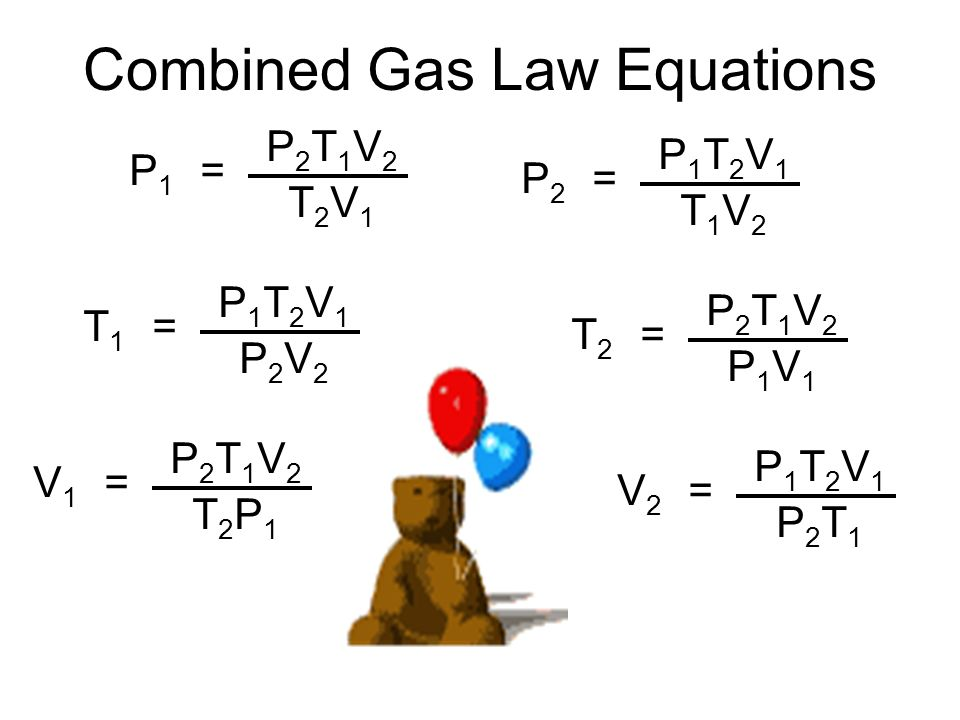# Combined Gas Law Study Guide

What is Combined Gas Law?

In everyday life, the combined gas law has applications. When the amount of gas remains constant, but the pressure, volume, and temperature fluctuate, this rule applies. Cloud formation, refrigerators, and air conditioners, for example, are all predicted by the law. It’s also employed in computations involving thermodynamics and fluid mechanics.

Pressure, temperature, and volume are all controlled by the combined gas law, which can be used to explain the mechanics.

The law describes the relationship between the pressure, volume, and temperature of a gas when the amount of gas is constant.

The Combined Gas Law equation is as follows:

(P1 × V1) / T1 = (P2 × V2) / T2

where P1 and P2 are the initial and final pressures of the gas, V1 and V2 are the initial and final volumes of the gas, and T1 and T2 are the initial and final temperatures of the gas in Kelvin.

### What is Combined Law Formula?

The combined gas law can be stated mathematically as

PV/T = k

Where,

P stands for pressure.

T = Kelvin temperature

V stands for volume.

K stands for constant (units of energy divided by temperature)

The law can be stated as follows when two chemicals are compared in two separate conditions:

P1V1/T1 = P2V2/T2

Where,

P2 stands for initial pressure.

Initial volume (V2)

T2 = temperature at the start

P1 stands for final pressure.

the final volume (V1)

T1 stands for the final temperature.

### Examples of Combined Gas Law

What is a practical application of the combined gas law? On the surface of the earth, a balloon filled with helium will have a specific pressure, temperature, and volume. If you let go of the balloon, it will soar. The temperature and air pressure start to diminish as you get higher in the air.

### Combined Gas Law EquationsAs an illustration

The gas has a 5L initial volume and a 3L final volume. Given an initial temperature of 273 K, a final temperature of 200 K, and a starting pressure of 25 kPa, calculate the gas’s final pressure.

Solution:

P2=25 kPa according to the specified parameters

V1 = 3L, V2 = 5L

273K = T1

T2 = 200 kelvin

P2V2/T2 = P1V1/T1, according to the combined gas law.

We get 25 x 5 / 273 = P1 x 3 / 200 by substituting in the formula.

As a result, P1 = 30.525 kPa.

# SUMMARY

• The combined gas law can be stated mathematically as: PV/T = k
• When everything except temperature, pressure, and volume are held constant, it is essentially a mixture of the other gas laws.
• In everyday life, the combined gas law has many applications.
• It’s also employed in computations involving thermodynamics and fluid mechanics.

## FAQ’s

Q. What is the combined gas law Formula?

PV/T = K is the formula for the combined gas law, where P denotes pressure, T denotes temperature, V denotes volume, and K denotes constant.

Q. What does combined gas law allow?

The combined gas law expresses the relationship between a fixed amount of gas’s pressure, volume, and absolute temperature.

Q. What stays the same in the combined gas law?

Only the amount of gas is kept constant in a combined gas law problem.

Q. Does combined gas law have to be in Kelvin?

Temperatures must be given in Kelvin when dealing with all gas laws.

We hope you enjoyed studying this lesson and learned something cool about Combined Gas Law! Join our Discord community to get any questions you may have answered and to engage with other students just like you! We promise, it makes studying much more fun😎

]]>